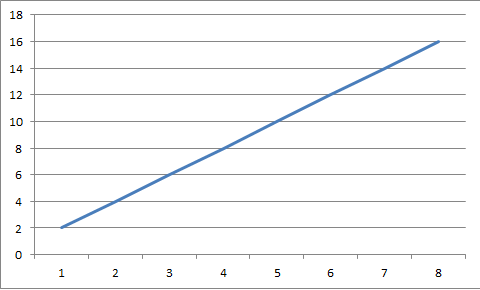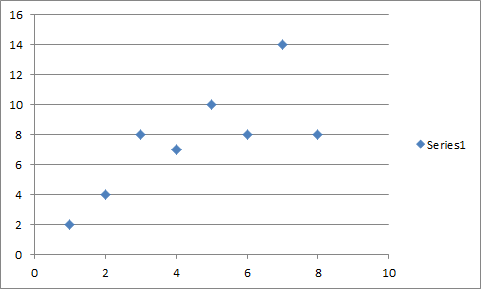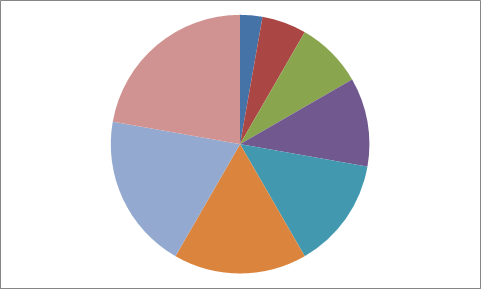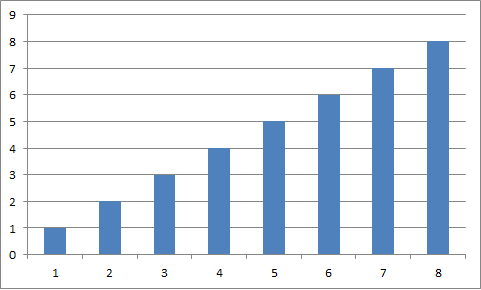Explore BrainMass

# Drawing Graphs

Graphs are a representation of a set of data where pairs of data are connects by links. It is an area of mathematics and computer science combining methods from geometric graph theory and information visualization to derive two-dimensional depictions of graphs. It is a pictorial representation of the vertices and edges of a graph.

Graphs measure many different qualities in an attempt to find objective means of evaluation their aesthetics and usability. The crossing number of a drawing is the number of pairs of edges that cross each other. If the graph is planar, it is often convenient to draw it without any edge intersections. The area of a drawing is the size of its smallest bounding box, relative to the closest distance between any two vertices. Symmetry display is the problem of finding symmetry groups within a given graph, and finding a drawing that displays as much of the symmetry as possible. The slope number of a graph is the minimum number of distinct edge slopes needed in a drawing with straight line segment edges.

There are many different types of graphs; there are simple bar graphs to more complex box and whisker plots. Drawing graphs with an x- and y-axis requires an independent and dependant variable. The dependant variable goes on the y-axis and the independent variable goes on the x-axis, such as time and distance. It is important to maintain the same scale throughout the graph.Line GraphScatter PlotPie ChartBar Graph

© BrainMass Inc. brainmass.com April 15, 2021, 7:00 pm ad1c9bdddf

## BrainMass Solutions Available for Instant Download

### Motion Supplies Sets

1. A truck filled with supplies sets out across the country at 9:00 A.M. It is traveling at a constant speed of 80.0 km/h. Two hours later, a train starts out from the same location with similar supplies going to the same destination. The train is traveling at a constant speed of 110 km/h. a. On the same axis, draw the posit

### Illustrate the U.S wholesale market for roses.

Wholesalers of roses (the firms that supply your local flower shop with roses for Valentine's Day) buy and sell roses in containers that hold 120 stems. The table provides information about the wholesale market for roses in the United States. The demand schedule is the wholesaler's demand and the supply schedule is the U.S. rose

### Graph/Limit of sin(x)/x, Graph of tangent of sin(x)

1) The limit of f(x) = (sin x)/x as x approaches 0 is 1. a) Use a graphing utility to graph the function f on the interval (- pi (<=) 0 (<=) pi ) (meaning negative pi is less than or equal to zero which is less than or equal to pi) Explain how the graph helps confirm the theorem. b) Explain how you could use a table o

### Draw the graph of the following parabolas

Draw the graph of the following parabolas: 1. 4y² = -3x 2. x² = 7y 3. (y - 3)² = 12(x + 2) 4. (x + 4)² = -40y 5. x² = 12(y - 2) 6. y² = -32 (x + 3) 7. (x + 1/2)² - 3/2y = 0 8. (x + 4)² = 8(y - 6) 9. V(-3, 6) and F(-1, 6) 10. V(0,0), passing P(4, 6), and axis horizontal

### Math Graphing Template

Graphing Template attached separately (see file attachment). Short Answer Use the Math Graphing Template to complete the following questions. 1. Graph the data in the table below. Which kind of function best models the data? Write an equation to model the data. x y 0 30 1 6 2 1.2 3 0.24 4 0.048 2. Graph t

### Equations of Lines through a Point

46. Draw line h through (0,3) with slope 1 and line h through (0,0) with slope 1. 48. Draw h through (-2,11) with slope 2/3 and draw h through (-2,1) with slope -3/2. 58. In each case determine weather the lines h and h are parallel , perpendicular or neither a) Line h goes through (-1,4) and (4,6). Line h goes throu

### Graphing by using Corresponding y-Values from Random x-Values

I am having trouble understanding how to choose an x value and a corresponding y value, for example, y= -3x + 1, y= -4/5x + 13/7, or y=4 - 3x -x^2 Please explain how you choose a random x,y when graphing

### Draw the graph of each line by using its y-intercept and its slope.

Draw the graph of each line using its y-intercept and its slope. 4x + 3y = 9

### Graphing Appropriate Boxes

1. I need to indicate by checking the appropriate box, whether the given points are on line 1, or line 2, or both line 1 and line 2, or on neither one of the two lines: LINE 1: 3x-4y-20=0 LINE 2: 8x+3y+15=0 (x,y) Line 1 line 2 Both lines neither line (0,-5) (-3,3) (4,-2) 2. Using the va

### How do you draw an xy graph?

How do you draw an xy graph?